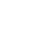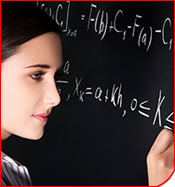### MAT-101COLLEGE ALGEBRA 3 Credits

This course is a part of:
Associate's Degree Program
Bachelor's Degree Program

This course is designed to introduce the student to the basic principles of algebra. These principles include mathematical expressions such as polynomials, exponentials, and logarithms, and their manipulations. We will also cover functions and their graphs. The course will discuss a wide variety of equations such as inequalities, linear equations and quadratic equations and their solutions through algebra and graphing. Graphing of more complex equations such as parabolas, hyperbolas and circles will also be covered.

## Course Learning Outcomes

By the end of the course, the student will be able to:

• Use mathematical notations and expressions to represent variables, and write a algebraic expressions and equations.
• Solve algebraic equations, including equations that are linear, quadratic, involve polynomials, roots, and rational functions.
• Graph a mathematical function and apply basic transformations to the graph and to transform the equation correspondingly.
• Work with and manipulate exponential and logarithmic expressions.
• Solve systems of linear and basic nonlinear equations, and find the solution sets of systems of inequalities.
• Recognize equations that represent conic sections such as circles, ellipses, hyperbolas, and parabolas from mathematic equations and their graphs representations.